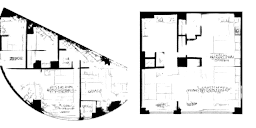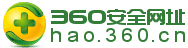#0431-85102283#### 热门资讯

##### 联系我们#### 代码详解：一文读懂自动编码器的前世今生· 潜在空间中的间隙

· 潜在空间中的可分性

· 离散潜在空间

MNIST数据集的潜在空间表示

Fashion MNIST

## Download the data(x_train, y_train), (x_test, y_test) = datasets.fashion_mnist.load_data()## normalize and reshapex_train = x_train/255.x_test = x_test/255.x_train = x_train.reshape(-1, 28, 28, 1)x_test = x_test.reshape(-1, 28, 28, 1)# Lets add sample noise - Salt and Peppernoise = augmenters.SaltAndPepper(0.1)seq_object = augmenters.Sequential([noise])train_x_n = seq_object.augment_images(x_train * 255) / 255val_x_n = seq_object.augment_images(x_test * 255) / 255

# input layerinput_layer =Input(shape=(28, 28, 1))# encodingarchitectureencoded_layer1= Conv2D(64, (3, 3), activation='relu', padding='same')(input_layer)encoded_layer1= MaxPool2D( (2, 2), padding='same')(encoded_layer1)encoded_layer2= Conv2D(32, (3, 3), activation='relu', padding='same')(encoded_layer1)encoded_layer2= MaxPool2D( (2, 2), padding='same')(encoded_layer2)encoded_layer3= Conv2D(16, (3, 3), activation='relu', padding='same')(encoded_layer2)latent_view = MaxPool2D( (2, 2),padding='same')(encoded_layer3)# decodingarchitecturedecoded_layer1= Conv2D(16, (3, 3), activation='relu', padding='same')(latent_view)decoded_layer1= UpSampling2D((2, 2))(decoded_layer1)decoded_layer2= Conv2D(32, (3, 3), activation='relu', padding='same')(decoded_layer1)decoded_layer2= UpSampling2D((2, 2))(decoded_layer2)decoded_layer3= Conv2D(64, (3, 3), activation='relu')(decoded_layer2)decoded_layer3= UpSampling2D((2, 2))(decoded_layer3)output_layer = Conv2D(1, (3, 3), padding='same',activation='sigmoid')(decoded_layer3)# compile themodelmodel =Model(input_layer, output_layer)model.compile(optimizer='adam',loss='mse')# run themodelearly_stopping= EarlyStopping(monitor='val_loss', min_delta=0, patience=10, verbose=5,mode='auto')history =model.fit(train_x_n, x_train, epochs=20, batch_size=2048,validation_data=(val_x_n, x_test), callbacks=[early_stopping])input_layer= Input(shape=(258, 540, 1))#encoderencoder= Conv2D(64, (3, 3), activation='relu', padding='same')(input_layer)encoder= MaxPooling2D((2, 2), padding='same')(encoder)#decoderdecoder= Conv2D(64, (3, 3), activation='relu', padding='same')(encoder)decoder= UpSampling2D((2, 2))(decoder)output_layer= Conv2D(1, (3, 3), activation='sigmoid', padding='same')(decoder)ae =Model(input_layer, output_layer)ae.compile(loss='mse',optimizer=Adam(lr=0.001))batch_size= 16epochs= 200early_stopping= EarlyStopping(monitor='val_loss',min_delta=0,patience=5,verbose=1,mode='auto')history= ae.fit(x_train, y_train, batch_size=batch_size, epochs=epochs,validation_data=(x_val, y_val), callbacks=[early_stopping])

batch_size = 16latent_dim = 2 # Number of latent dimension parameters# ENCODER ARCHITECTURE: Input -> Conv2D*4 -> Flatten -> Denseinput_img = Input(shape=(28, 28, 1))x = Conv2D(32, 3,padding='same',activation='relu')(input_img)x = Conv2D(64, 3,padding='same',activation='relu',strides=(2, 2))(x)x = Conv2D(64, 3,padding='same',activation='relu')(x)x = Conv2D(64, 3,padding='same',activation='relu')(x)# need to know the shape of the network here for the decodershape_before_flattening = K.int_shape(x)x = Flatten()(x)x = Dense(32, activation='relu')(x)# Two outputs, latent mean and (log)variancez_mu = Dense(latent_dim)(x)z_log_sigma = Dense(latent_dim)(x)## SAMPLING FUNCTIONdef sampling(args):z_mu, z_log_sigma = args epsilon = K.random_normal(shape=(K.shape(z_mu), latent_dim),mean=0., stddev=1.)return z_mu + K.exp(z_log_sigma) * epsilon# sample vector from the latent distributionz = Lambda(sampling)([z_mu, z_log_sigma])## DECODER ARCHITECTURE# decoder takes the latent distribution sample as inputdecoder_input = Input(K.int_shape(z)[1:])# Expand to 784 total pixelsx = Dense(np.prod(shape_before_flattening[1:]),activation='relu')(decoder_input)# reshapex = Reshape(shape_before_flattening[1:])(x)# use Conv2DTranspose to reverse the conv layers from the encoderx = Conv2DTranspose(32, 3,padding='same',activation='relu',strides=(2, 2))(x)x = Conv2D(1, 3,padding='same',activation='sigmoid')(x)# decoder model statementdecoder = Model(decoder_input, x)# apply the decoder to the sample from the latent distributionz_decoded = decoder(z)

# construct a custom layer to calculate the lossclass CustomVariationalLayer(Layer):def vae_loss(self, x, z_decoded):x = K.flatten(x)z_decoded = K.flatten(z_decoded)# Reconstruction lossxent_loss = binary_crossentropy(x, z_decoded)# KL divergencekl_loss = -5e-4 * K.mean(1 + z_log_sigma - K.square(z_mu) - K.exp(z_log_sigma), axis=-1)return K.mean(xent_loss + kl_loss)# adds the custom loss to the classdef call(self, inputs):x = inputsz_decoded = inputsloss = self.vae_loss(x, z_decoded)self.add_loss(loss, inputs=inputs)return x# apply the custom loss to the input images and the decoded latent distribution sampley = CustomVariationalLayer()([input_img, z_decoded])# VAE model statementvae = Model(input_img, y)vae.compile(optimizer='rmsprop', loss=None)vae.fit(x=train_x, y=None,shuffle=True,epochs=20,batch_size=batch_size,validation_data=(val_x, None))

#### 相关产品